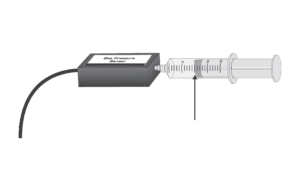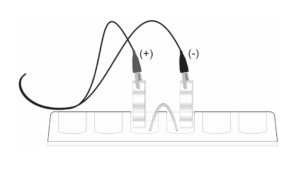# Chemistry

## Make Your Chemistry Classes More Elemental

Whether you are teaching Beer’s Law or exploring how humans use food for energy, Vernier technology and investigations will help your students better understand important chemistry concepts. Give your students insight into this vital subject with interactive learning opportunities from Vernier. With Vernier technology, you can engage students and foster a lifelong love of learning.

## Chemistry Product Categories

### Lab Books

#### Chemistry Resources

•Training​
•Questions? Contact us

#### We are chemists, educators, and your team

We’re here to support you as an educator as you integrate data-collection technology into your teaching. See how our products provide you with affordable classroom and laboratory solutions designed for student success.## Featured Chemistry Experiments### Beer’s Law Investigations

The primary objective of this Preliminary Activity is to determine the concentration of an unknown copper (II) sulfate solution. You will use a Colorimeter (a side view is shown above). In this device, red light from the LED light source will pass through the solution and strike a photocell. The CuSO4 solution used in this experiment is blue. A higher concentration of the colored solution absorbs more light (and transmits less) than a solution of lower concentration. The Colorimeter monitors the light received by the photocell as percent transmittance.

You will prepare five copper (II) sulfate solutions of known concentration (standard solutions). Each solution is transferred to a small, rectangular cuvette that is placed into the Colorimeter. The amount of light that penetrates the solution and strikes the photocell is used to compute the absorbance of each solution. When you graph absorbance vs. concentration for the standard solutions, a direct relationship should result. The direct relationship between absorbance and concentration for a solution is known as Beer’s law.### Exploring the Properties of Gases

The purpose of this investigation is to conduct a series of experiments, each of which illustrates a different gas law. You will be given a list of equipment and materials and some general guidelines to help you get started with each experiment. Four properties of gases will be investigated: pressure, volume, temperature, and number of molecules. By assembling the equipment, conducting the appropriate tests, and analyzing your data and observations, you will be able to describe the gas laws, both qualitatively and mathematically.### Electrochemistry: Voltaic Cells

In electrochemistry, a voltaic cell is a specially prepared system in which an oxidation-reduction reaction occurs spontaneously. This spontaneous reaction produces an easily measured electrical potential. Voltaic cells have a variety of uses.

In this experiment, you will prepare a variety of semi-microscale voltaic cells in a 24-well test plate. A voltaic cell is constructed by using two metal electrodes and solutions of their respective salts (the electrolyte component of the cell) with known molar concentrations. In Parts I and II of this experiment, you will use a Voltage Probe to measure the potential of a voltaic cell with copper and lead electrodes. You will then test two voltaic cells that have unknown metal electrodes and, through careful measurements of the cell potentials, identify the unknown metals. In Part III of the experiment, you will measure the potential of a special type of voltaic cell called a concentration cell. In the first concentration cell, you will observe how a voltaic cell can maintain a spontaneous redox reaction with identical copper metal electrodes, but different electrolyte concentrations. You will then measure the potential of a second concentration cell and use the Nernst equation to calculate the solubility product constant, Ksp, for lead iodide, PbI2.

### The Determination of an Equilibrium Constant

Chemical reactions occur to reach a state of equilibrium. The equilibrium state can be characterized by quantitatively defining its equilibrium constant, Keq. In this experiment, you will determine the value of Keq for the reaction between iron (III) ions and thiocyanate ions, SCN.${\text{F}}{{\text{e}}^{{\text{3}} + }}\left( {{\text{aq}}} \right) + {\text{ SC}}{{\text{N}}^-}\left( {{\text{aq}}} \right) \to {\text{FeSC}}{{\text{N}}^{{\text{2}} + }}\left( {{\text{aq}}} \right)$

The equilibrium constant, Keq, is defined by the equation shown below.${K_{eq}} = \frac{{[{\text{FeSC}}{{\text{N}}^{2 + }}]}} {{[{\text{F}}{{\text{e}}^{3 + }}][{\text{SC}}{{\text{N}}^ - }]}}$

To find the value of Keq, which depends only upon temperature, it is necessary to determine the molar concentration of each of the three species in solution at equilibrium. You will determine the concentration by measuring light that passes through a sample of the equilibrium mixtures. The amount of light absorbed by a colored solution is proportional to its concentration. The red FeSCN2+ solution absorbs blue light, thus the Colorimeter users will be instructed to use the 470 nm (blue) LED. Spectrometer users will determine an appropriate wavelength based on the absorbance spectrum of the solution.

## Featured Software and Digital Curriculum

Vernier Graphical Analysis™ Pro

With the new Graphical Analysis™ Pro app, users can insert, view, and sync a video to sensor data for inspection and analysis. This app is perfect for engaging students—either remotely or in the lab—in more advanced analysis of data from biology, chemistry, and physics experiments.

Lt for Chemistry covers core concepts in first year undergraduate introductory chemistry. The collection introduces a variety of concepts fundamental to chemistry, including acid-base titration, states of matter, heats of reaction, synthesis, buffers, and equilibria principles.

### Pivot Interactives

Pivot Interactives is a powerful supplement to hands‑on experimentation, enabling students to vary experimental parameters one at a time to view results from a set of many recordings of the same experiment.

• ### Go Direct® Temperature Probe

$75.00$659.00